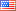# Revision history [back]

### I need to plot two differential equations on the same set of axes (with a legend showing which is which). My domain has to be 0≤t≤100. Listed below is my code, any help is greatly appreciated.

t = var('t') x = function('x')(t) y = function('y')(t) de1 = diff(x,t) == -3/20x + 1/12y de2 = diff(y,t) == 3/20x-3/20y sol = desolve_system([de1,de2],[x,y],ics=[0,4,0])

f(t) = sol.rhs() g(t) = sol.rhs()2 NoneJohn Palmieri6607 ●18 ●57 ●146 http://www.math.washin...

### I need to plot two differential equations on the same set of axes (with a legend showing which is which). My domain has to be 0≤t≤100. Listed below is my code, any help is greatly appreciated.

Listed below is my code:

t = var('t')
x = function('x')(t)
y = function('y')(t)
de1 = diff(x,t) == -3/20x -3/20*x + 1/12y
1/12*y
de2 = diff(y,t) == 3/20x-3/20y
3/20*x-3/20*y
sol = desolve_system([de1,de2],[x,y],ics=[0,4,0])desolve_system([de1,de2],[x,y],ics=[0,4,0])
f(t) = sol.rhs()
g(t) = sol.rhs()sol.rhs()3 None

### I need to plot two differential equations on the same set of axes (with a legend showing which is which). My domain has to be 0≤t≤100. Listed below is my code, any help is greatly appreciated.

Listed below is my code:

t = var('t')
x = function('x')(t)
y = function('y')(t)
de1 = diff(x,t) == -3/20*x + 1/12*y
de2 = diff(y,t) == 3/20*x-3/20*y
sol = desolve_system([de1,de2],[x,y],ics=[0,4,0])

f(t) = sol.rhs()
g(t) = sol.rhs()4 None

### Plot solutions of two differential equations on same axes with legend

I need to plot two differential equations on the same set of axes axes (with a legend showing which is which). which).

My domain has to be 0≤t≤100. 0 ≤ t ≤ 100.

Listed below is my code, any help is greatly appreciated.

Listed below is my code:

t = var('t')
x = function('x')(t)
y = function('y')(t)
de1 = diff(x,t) == -3/20*x + 1/12*y
de2 = diff(y,t) == 3/20*x-3/20*y
sol = desolve_system([de1,de2],[x,y],ics=[0,4,0])

f(t) = sol.rhs()
g(t) = sol.rhs()